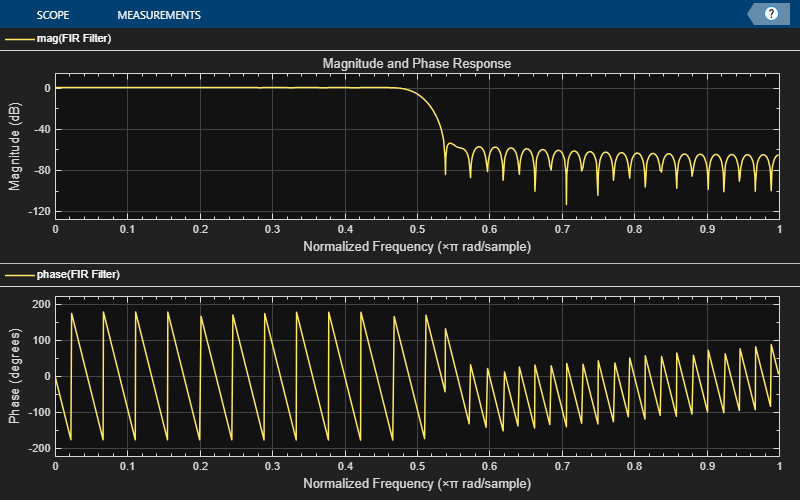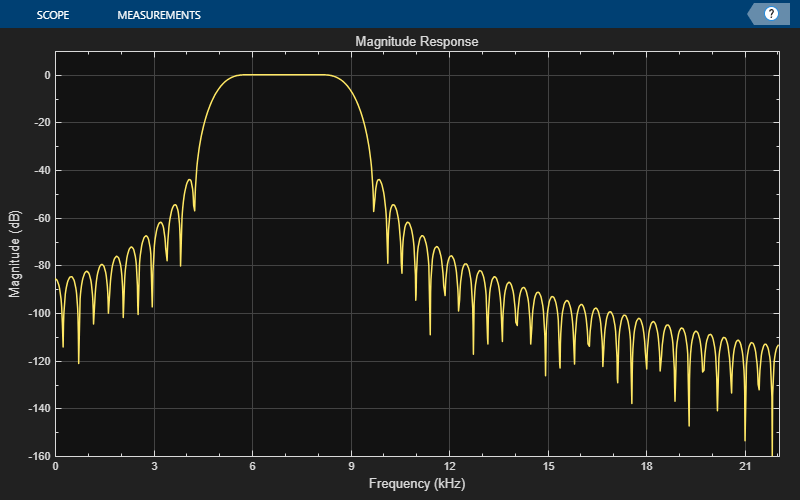step

Display time-varying magnitude response

Description

example

step(dfv,filt) displays the time-varying magnitude response of the object filter, filt, in the Dynamic Filter Visualizer figure, as long as filt has a valid freqz() implementation.

example

step(dfv,B,A) displays the magnitude response for the digital filters with numerator and denominator polynomial coefficients stored in B1 and A1, B2 and A2, ..., and BN and AN, respectively.

Examples

collapse all

Design an FIR filter with time-varying magnitude response. Plot this varying response on a dynamic filter visualizer.

Create a dsp.DynamicFilterVisualizer object.

dfv = dsp.DynamicFilterVisualizer('YLimits',[-120 10])
dfv =
DynamicFilterVisualizer with properties:

FFTLength: 2048
SampleRate: 44100
FrequencyRange: [0 22050]
XScale: 'Linear'
MagnitudeDisplay: 'Magnitude (dB)'

Visualization
Name: 'Dynamic Filter Visualizer'
Title: 'Magnitude Response'
YLimits: [-120 10]
ShowLegend: 0
FilterNames: {''}
Position: [240 262 800 500]

Vary the cutoff frequency of the FIR filter, k, from 0.1 to 0.5 in increments of 0.001. View the varying magnitude response using the dynamic filter visualizer.

for k = 0.1:0.001:0.5
b = fir1(90,k);
dfv(b,1);
endVisualize the varying magnitude response of the variable bandwidth FIR filter using the dyamic filter visualizer.

Create a dsp.DynamicFilterVisualizer object.

dfv = dsp.DynamicFilterVisualizer('YLimits',[-160 10])
dfv =
DynamicFilterVisualizer with properties:

FFTLength: 2048
SampleRate: 44100
FrequencyRange: [0 22050]
XScale: 'Linear'
MagnitudeDisplay: 'Magnitude (dB)'

Visualization
Name: 'Dynamic Filter Visualizer'
Title: 'Magnitude Response'
YLimits: [-160 10]
ShowLegend: 0
FilterNames: {''}
Position: [240 262 800 500]

Design a bandpass variable bandwidth FIR filter with a center frequency of 5 kHz and a bandwidth of 4 kHz.

Fs = 44100;
vbw = dsp.VariableBandwidthFIRFilter('FilterType','Bandpass',...
'FilterOrder',100,...
'SampleRate',Fs,...
'CenterFrequency',5e3,...
'Bandwidth',4e3);

Vary the center frequency of the filter. Visualize the varying magnitude response of the filter using the dsp.DynamicFilterVisualizer object.

for idx  = 1:100
dfv(vbw);
vbw.CenterFrequency = vbw.CenterFrequency + 20;
endInput Arguments

collapse all

Dynamic filter visualizer, specified as a dsp.DynamicFilterVisualizer object.

Filter System object with a valid freqz() implementation.

Numerator polynomial coefficients, specified as a row vector.

Data Types: single | double

Denominator polynomial coefficients, specified as a:

• scalar –– The filter is an FIR filter.

• row vector –– The filter is an IIR filter.

Data Types: single | double

Objects

Introduced in R2018b

DSP System Toolbox DocumentationGet trial now